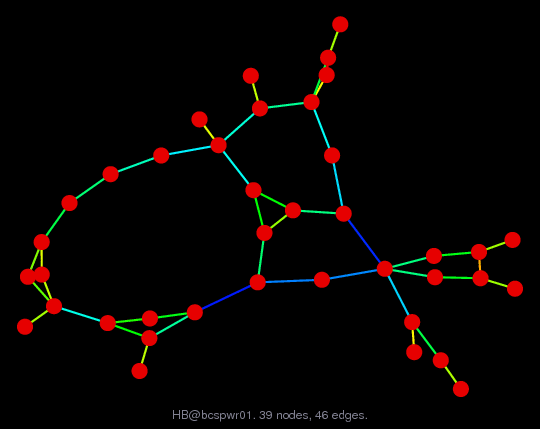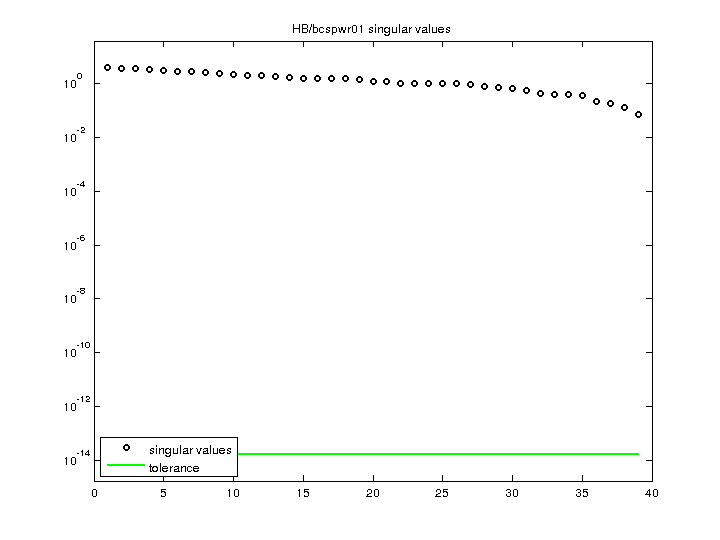Matrix: HB/bcspwr01

Description: SYMMETRIC STRUCTURE (STANDARD TEST POWER SYSTEM - NEW ENGLAND)(undirected graph drawing)• Matrix group: HB
• download as a MATLAB mat-file, file size: 711 bytes. Use UFget(13) or UFget('HB/bcspwr01') in MATLAB.

 Matrix properties number of rows 39 number of columns 39 nonzeros 131 structural full rank? yes structural rank 39 # of blocks from dmperm 1 # strongly connected comp. 1 explicit zero entries 0 nonzero pattern symmetry symmetric numeric value symmetry symmetric type binary structure symmetric Cholesky candidate? yes positive definite? no

 author B. Dembart, J. Lewis editor I. Duff, R. Grimes, J. Lewis date 1981 kind power network problem 2D/3D problem? no

 Ordering statistics: result nnz(chol(P*(A+A'+s*I)*P')) with AMD 104 Cholesky flop count 2.9e+02 nnz(L+U), no partial pivoting, with AMD 169 nnz(V) for QR, upper bound nnz(L) for LU, with COLAMD 111 nnz(R) for QR, upper bound nnz(U) for LU, with COLAMD 194

 SVD-based statistics: norm(A) 3.83636 min(svd(A)) 0.0730734 cond(A) 52.5001 rank(A) 39 sprank(A)-rank(A) 0 null space dimension 0 full numerical rank? yes

 singular values (MAT file): click here SVD method used: s = svd (full (A)) status: ok